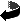# Positive mass theorem for the Yamabe problem on spin manifolds by Bernd Ammann, Emmanuel Humbert

Positive mass theorem for the Yamabe problem on spin manifolds .pdf
GAFA 15 (2005), 567-576.

### Abstract

Let $(M,g)$ be a compact connected spin manifold of dimension $n\geq 3$ whose Yamabe invariant is positive. We assume that $(M,g)$ is locally conformally flat or that $n \in \{3,4,5\}$. According to a positive mass theorem of Witten, the constant term in the asymptotic development of the Green's function of the conformal Laplacian is positive if $(M,g)$ is not conformally equivalent to the sphere. Using Witten's argument, we give a very short proof of this fact. This simplifies considerably the proof of the Yamabe problem for spin manifolds.

### Mathematics Subject Classification

53C21 (Primary), 58E11, 53C27 (Secondary)

### Erratum

In the printed version a term in a local development of the Dirac operator is not complete, a term is missing.
Here is the corrected version: .pdf
Here is the preprint version close to the printed version: .dvi, .ps,.ps.gz oder .pdf

### Keywords

Positive mass theorem, Yamabe problem, spin manifoldsZurück zur Homepage

Bernd Ammann, Emmanuel Humbert,
The Paper was written on 01.04.2003
Last update of the www-page 3.2.2019